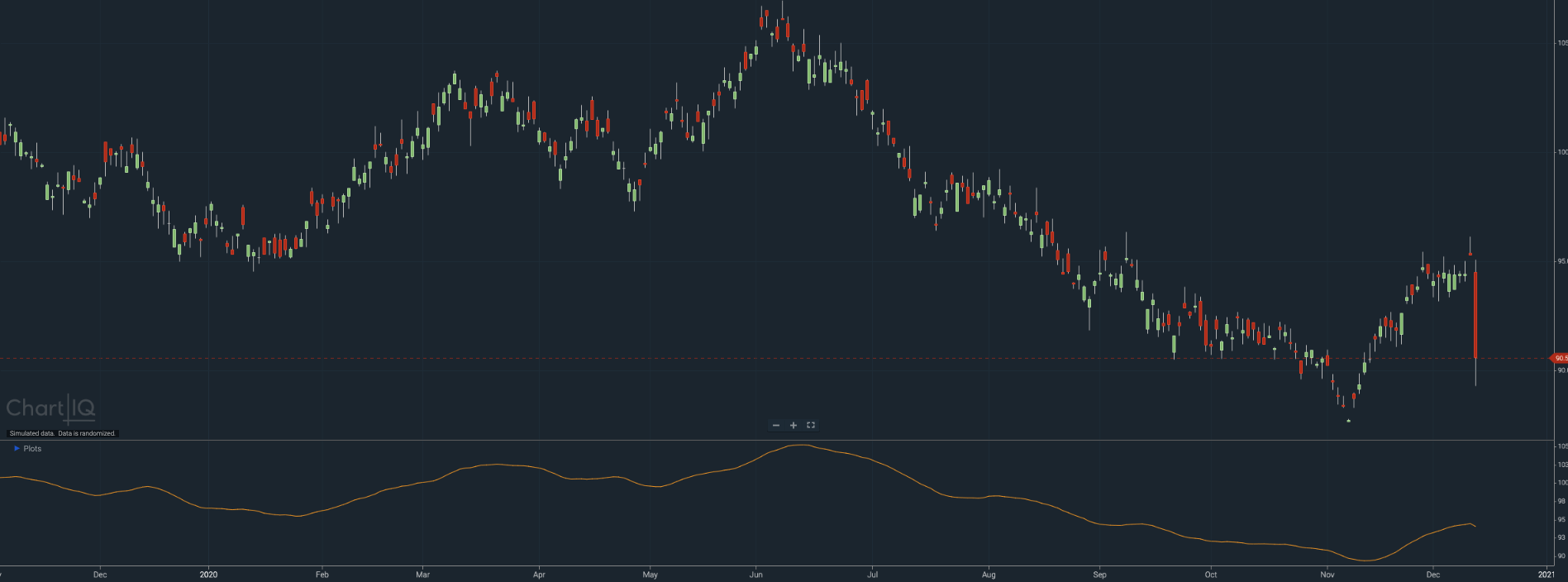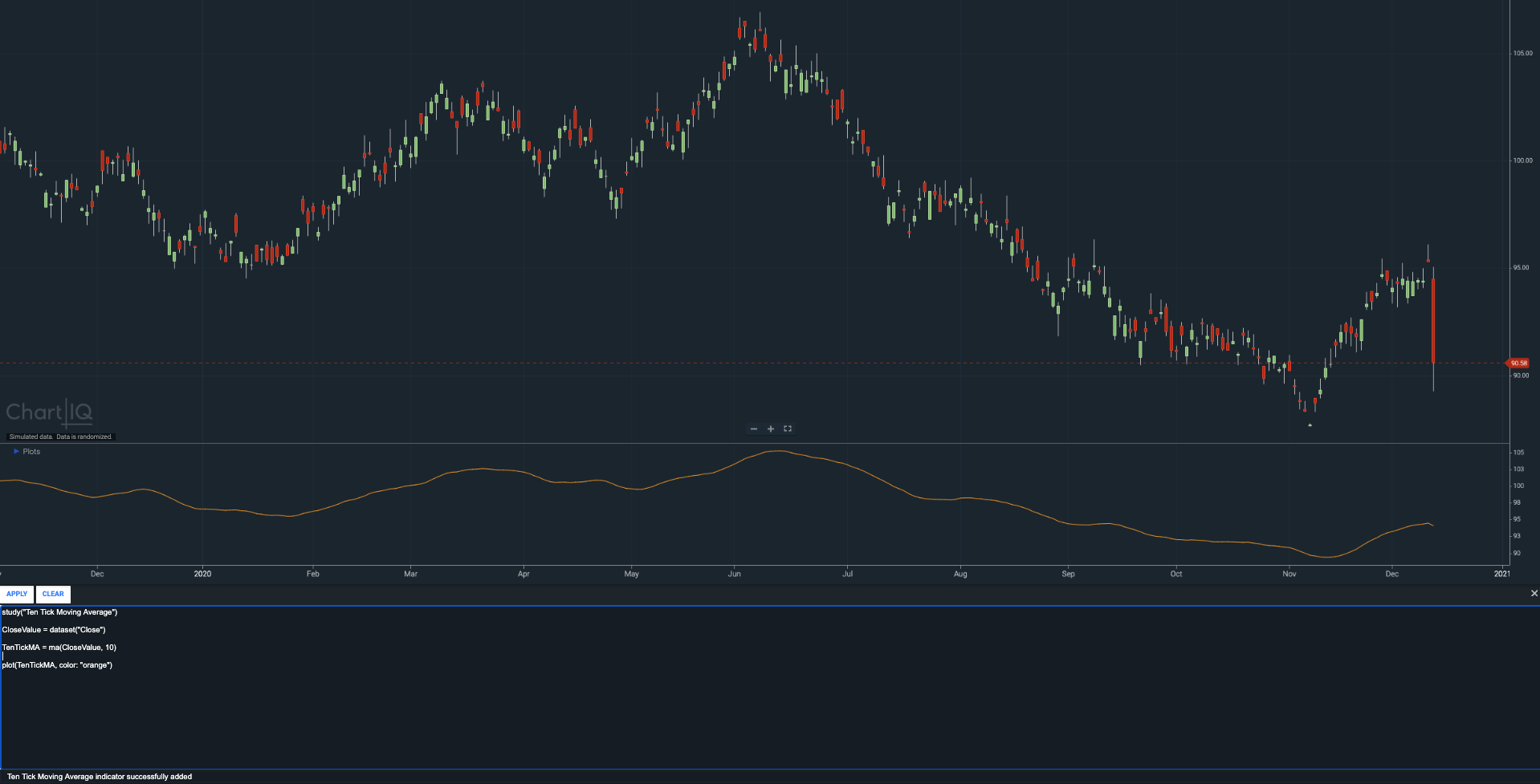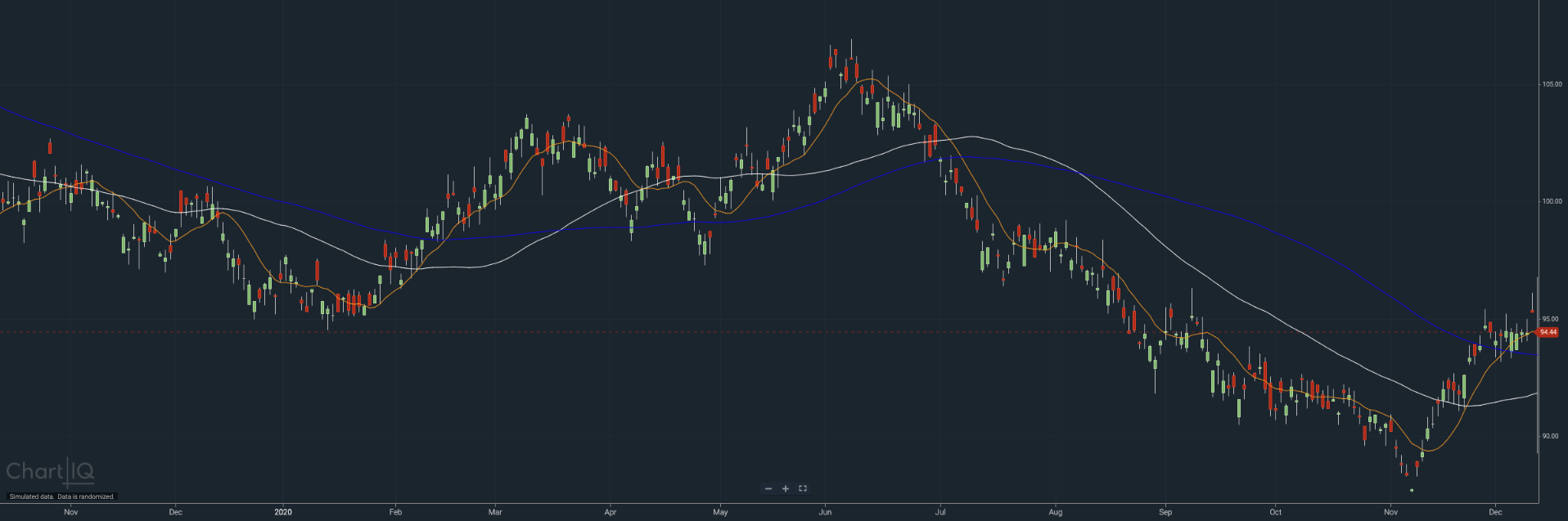# Recipe 3: Moving Averages

Cookbook Home

What do we want to see?

A simple moving average line that can be based with any average number of data ticks:How do I use ScriptIQ to draw a moving average of the last 10 tick close values? (2 steps, if building on Recipe 2)

1. From Recipe 2 you should understand the following example:

``````study("Close Value Line")
# This is how and where you name the indicator.

CloseValue = dataset("Close")
# This shows how we grab the "close value" from the hidden data set for each tick.

plot(CloseValue)
# This draws your line between each "close value" for each date along the x-axis.

# And the # symbol is how I place notes here for
# you so that if you copy and paste my notes into the ScriptIQ panel,
# then they will not break your indicator. :)

# Feel free to delete any line that starts with a #.
``````

Now after `CloseValue = dataset("Close")`, add `TenTickMA = ma(Your Variable Name Here, 10)` and rename your study — maybe use the following: `study("Ten Tick Moving Average")`.

You should have something like below:

``````study("Ten Tick Moving Average")

CloseValue = dataset("Close")

TenTickMA = ma(CloseValue, 10)
# New line of code just above. :)

plot(CloseValue)
``````
2. Now replace the variable `CloseValue` in `plot(CloseValue)` with `TenTickMA`, and give your plot a color.

You should have something like the following:

``````study("Ten Tick Moving Average")

CloseValue = dataset("Close")

TenTickMA = ma(CloseValue, 10)
# New line of code just above. :)

plot(TenTickMA, color: "orange")
``````

Click and hold for 2 seconds on your new orange "Ten Tick Moving Average" line and drag it onto your main chart.

Congratulations, you have just drawn your Moving Average indicator!## Recipe 3 Secret

`ma(___,any number you pick)` can have any length of number to create a moving average. When you combine this with multiple moving averages that have a different period of time (length of numbers), then you can look for moving average crosses.

For instance:

``````study("10, 50, and 100 Tick Moving Averages")

CloseValue = dataset("Close")

TenTickMA = ma(CloseValue, 10)

FiftyTickMA = ma(CloseValue, 50)

OneHundredTickMA = ma(CloseValue, 100)

plot(TenTickMA, color: "orange")
plot(FiftyTickMA, color: "white")
plot(OneHundredTickMA, color: "blue")
``````

and you should get the following:## Recap

• 2 steps learned to create a moving average
• 1 secret learned to modify the moving average's periods for the average and to draw multiple moving averages

At this point take the leap and start reading the developer's documentation. If you understood this recipe, then we are confident you will understand how to use the additional moving average tools in the ScriptIQ API developer's documentation.

Cookbook Home